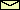# The Pendulum with Rotating Suspension Point

acceleration of gravity = 9.81 m/sec2

For instructions click on here.
For the equation of motion click on here

Suggestions for EXPERIMENTS
1. Choose length = 1 m, damping = 1 sec-1, and amplitude = 0.2 m (this are the default settings when the applet is started). Now, change the frequency of driving very slowly from 0.2 Hz to 0.8 Hz. Observe the change in the amplitude of oscillation and the change in the phase between driving and pendulum oscillations. You can measure it by using the oscilloscope.
 Related topics in the lecture room: Resonance.
2. Choose length = 1 m, damping = 0.1 sec-1, amplitude = 0.07 m, and frequency = 1.2 Hz. Slowly decrease the frequency down to 0.2 Hz and observe (and measure) the amplitude of oscillation. You should find two regimes of strong oscillations (resonances). In order to distinguish both regimes, compare the oscillation frequency with the driving frequency.
 Related topics in the lecture room: Parametric resonance.
3. Choose length = 1 m, damping = 0.1 sec-1, amplitude = 0.07 m, and frequency = 0.44 Hz. Depending on the initial condition, you will get either a strong or a weak oscillation.
 Related topics in the lecture room: Bistability and foldover effect.
4. Choose length = 1 m, damping = 0.1 sec-1, amplitude = 0.07 m, and frequency = 0.8 Hz. Depending on the initial condition, you will get either a strong or a weak oscillation.
 Related topics in the lecture room: Bistability and parametrically excited oscillations.
5. Choose length = 1 m, damping = 1 sec-1, and frequency = 0.7 Hz,. Slowly increase the amplitude of driving from 0.3 m to 1.8 m and observe the increase and decrease of complexity in the motion of the pendulum. For example, you will get period-doubling bifurcation between 0.35 m and 0.36 m, chaos at 0.4 m and 0.85 m, a period-4 orbit at 0.8 m, and bistability between 1.45 m and 1.7 m.
 Related topics in the lecture room: Nonlinear dynamics.

© 1998 Franz-Josef Elmer,Franz-Josef doht Elmer aht unibas doht ch, last modified Wednesday, June 27, 2011.